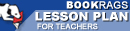Lesson Plans

# Journey Through Genius: The Great Theorems of Mathematics Quiz | Eight Week Quiz C

This set of Lesson Plans consists of approximately 142 pages of tests, essay questions, lessons, and other teaching materials.
 View a FREE sampleName: _________________________ Period: ___________________

This quiz consists of 5 multiple choice and 5 short answer questions through Heron's Formula for Triangular Area.

## Multiple Choice Questions

1. As described by Dunham, what did Archimedes demonstrate first in his proof on pi?
(a) Area of a circle is equal to that of a right triangle that has one leg equal to the circle's radius and the other leg equal to the circle's diameter.
(b) Area of a circle is equal to that of a right triangle that has one leg equal to the circle's radius and the other leg equal to the circle's circumference.
(c) Area of a circle is equal to that of a right triangle that has one leg equal to the circle's diameter and the other leg equal to the circle's circumference.
(d) Area of a circle is equal to that of a right triangle that has one leg equal to the circle's hypotenuse and the other leg equal to the circle's circumference.

2. Dunham showed that Heron's proof could also be used as which of the following?
(a) A proof of Hippocrates' squared areas.
(b) A proof of the Pythagorean Theorem.
(c) A proof of Euclid's number theory
(d) A proof of Archimedes' number theory.

3. After working on pi, what did Archimedes continue with in his study of mathematics?
(a) He studied the volume to surface area ratios of cubes.
(b) He studied the relationship between ratios in triangles.
(c) He studied the volume and surface area of spheres, cones, and cylinders.
(d) He studied the relationship of sine to cosine.

4. As described by Archimedes, what is always true about he diameter of the circle?
(a) It's never proportional to its circumference.
(b) It's equal to the square of the radius.
(c) It's equal to pi.
(d) It's always proportional to its circumference.

5. In general, what did Euclid's number theory describe?
(a) The relationship of decimals to integers.
(b) The nature of whole numbers.
(c) The nature of measuring geometry.
(d) The relationship of fractions to decimals.

## Short Answer Questions

1. Besides being a mathematician, what else other work was Archimedes famous for?

2. Heron's work referred to the work of what other famous scholar?

3. How did Archimedes arrive at a number value for pi?

4. Which of the following was one of Euclid's great theorems?

5. Which words best describe how solid proofs were developed in Elements?

(see the answer key)

 This section contains 451 words (approx. 2 pages at 300 words per page) View a FREE sampleCopyrightsJourney Through Genius: The Great Theorems of Mathematics from BookRags. (c)2019 BookRags, Inc. All rights reserved.
Follow Us on Facebook